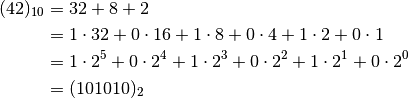# 16. Appendix¶

You really don’t know when to stop, do you?

## 16.1. Basic algebra¶

Let’s introduce some number theory. Any number can be represented by the following term: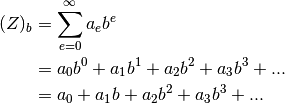Now, that was very abstract. Let’s explain first, then make some examples.

The first thing you need to know is the numbers sign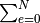. It’s just a shorthand notation for a sum of many terms. The terms can use a sum variablewhich starts from an initial value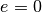and goes to some maximum value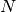. So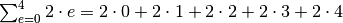.

We also use two exponential laws, namely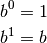Let’s make an example with base.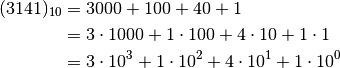Let’s now write the same number in the binary system.Since we’re dealing with computers, here’s an example with base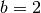. Let’s write the number 42 in binary.